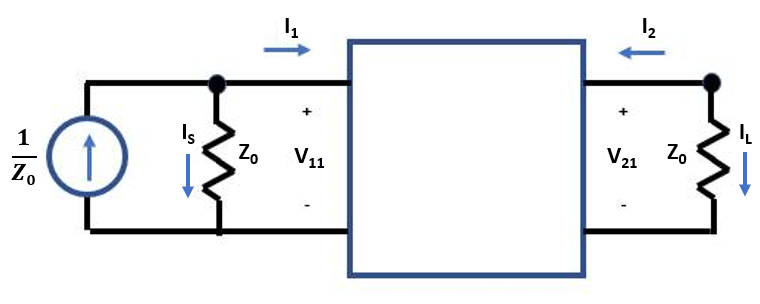Extract S-Parameters from Circuit

This example uses the Symbolic Math Toolbox to explain how the RF Toolbox extracts two-port S-parameters from an RF Toolbox circuit object.

Consider a two-port network as shown in the figure 1that you want to characterize with S-parameters. S-parameters are defined as $\mathit{V}\text{\hspace{0.17em}}\mathit{–}\text{\hspace{0.17em}}\mathit{I}×{\mathit{Z}}_{0}=\text{\hspace{0.17em}}\mathit{S}\left(\mathit{V}\text{\hspace{0.17em}}+\text{\hspace{0.17em}}\mathit{I}×{\mathit{Z}}_{0}\right)$.Figure 1: Two-Port Network

To extract the S-parameters from a circuit into an sparameters object, the RF Toolbox terminates each port with the reference impedance ${\mathit{Z}}_{0}$. Then, the RF Toolbox independently drives each port j, with $\frac{1}{{\mathit{Z}}_{0}}$ and solves for the port voltages ${\mathit{V}}_{\mathit{ij}}$. Driving with current sources is the Norton equivalent of driving with a 1 V source and a series resistance of ${\mathit{Z}}_{0}$.

Measure the port voltage ${\mathit{V}}_{\mathit{ij}}$ at node i when node j is driven.

• If i $\ne$ j, the S-parameter entry ${\mathit{S}}_{\mathit{ij}}$ is simply twice the port voltage ${\mathit{V}}_{\mathit{ij}}$, and this is given using the equation ${\mathit{S}}_{\mathit{ij}}=2×{\mathit{V}}_{\mathit{ij}}$.

• The diagonal entries of S-parameters when $\mathit{i}=\mathit{j}$ are given using the equation ${\mathit{S}}_{\mathit{ij}}=2×{\mathit{V}}_{\mathit{ij}}-1$.Figure 2: Circuit Driven at Port 1 with Current Source

Write Constitutive and Conservative Equations of Circuit

Circuits are represented in node-branch form in the RF Toolbox. There are four branches in the circuit represented in figure 2, one for the input port, two for the two-port nport object, and one for the output port. This means that the circuit has four branch current unknowns ${\mathit{I}}_{\mathit{S}}$, ${\mathit{I}}_{1}$, ${\mathit{I}}_{2}$, and ${\mathit{I}}_{\mathit{L}}$ and two node voltages ${\mathit{V}}_{11}$ and ${\mathit{V}}_{21}$. To represent the circuit described in figure 2 in node-branch form, you need four constitutive equations to represent the branch currents and two conservative equations to represent the node voltages.

syms F IS I1 I2 IL V1 V2 Z0
syms S11 S12 S21 S22

nI = 4; % number of branch currents
nV = 2; % number of node voltages

% F = [Fconstitutive; Fconservative]
F = [
V1 - Z0*IS
V1 - Z0*I1 - S11*(V1+Z0*I1) - S12*(V2+Z0*I2)
V2 - Z0*I2 - S21*(V1+Z0*I1) - S22*(V2+Z0*I2)
V2 - Z0*IL
IS+I1
I2+IL
]
F =

$\left(\begin{array}{c}{V}_{1}-\mathrm{IS} {Z}_{0}\\ {V}_{1}-{I}_{1} {Z}_{0}-{S}_{11} \left({V}_{1}+{I}_{1} {Z}_{0}\right)-{S}_{12} \left({V}_{2}+{I}_{2} {Z}_{0}\right)\\ {V}_{2}-{I}_{2} {Z}_{0}-{S}_{21} \left({V}_{1}+{I}_{1} {Z}_{0}\right)-{S}_{22} \left({V}_{2}+{I}_{2} {Z}_{0}\right)\\ {V}_{2}-\mathrm{IL} {Z}_{0}\\ {I}_{1}+\mathrm{IS}\\ {I}_{2}+\mathrm{IL}\end{array}\right)$

Jacobian Evaluation of Circuit

Use the jacobian function from the Symbolic Math Toolbox to compute the matrix of derivatives of the function F with respect to the six unknowns (four branch currents and two node voltages)

J = jacobian(F,[IS; I1; I2; IL; V1; V2])
J =

$\left(\begin{array}{cccccc}-{Z}_{0}& 0& 0& 0& 1& 0\\ 0& -{Z}_{0}-{S}_{11} {Z}_{0}& -{S}_{12} {Z}_{0}& 0& 1-{S}_{11}& -{S}_{12}\\ 0& -{S}_{21} {Z}_{0}& -{Z}_{0}-{S}_{22} {Z}_{0}& 0& -{S}_{21}& 1-{S}_{22}\\ 0& 0& 0& -{Z}_{0}& 0& 1\\ 1& 1& 0& 0& 0& 0\\ 0& 0& 1& 1& 0& 0\end{array}\right)$

Solve S-Parameters of Circuit

Create a two-column right-hand side vector, rhs, to represent the driving of each port.

syms rhs [nI+nV 2]
syms x v S

rhs(:,:) = 0;
rhs(nI+1,1) = 1/Z0;  % rhs for driving input port
rhs(nI+nV,2) = 1/Z0  % rhs for driving output port
rhs =

$\left(\begin{array}{cc}0& 0\\ 0& 0\\ 0& 0\\ 0& 0\\ \frac{1}{{Z}_{0}}& 0\\ 0& \frac{1}{{Z}_{0}}\end{array}\right)$

To solve for the voltages, back solve the rhs with the Jacobian. The S-parameter matrix that MATLAB outputs represents the two-port circuit shown in figure 1.

x = J \ rhs;
v = x(nI+[1 nV],:);
S = 2*v - eye(2)
S =

$\left(\begin{array}{cc}{S}_{11}& {S}_{12}\\ {S}_{21}& {S}_{22}\end{array}\right)$